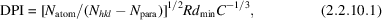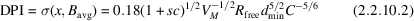International
Tables for
Crystallography
Volume F
Crystallography of biological macromolecules
Edited by E. Arnold, D. M. Himmel and M. G. Rossmann

International Tables for Crystallography (2012). Vol. F, ch. 2.2, p. 73

## Section 2.2.10. Error estimation for the refined model

H. M. Einspahra* and M. S. Weissb

aPO Box 6483, Lawrenceville, NJ 08648–0483, United States, and bHelmholtz-Zentrum Berlin für Materialien und Energie, Macromolecular Crystallography (HZB-MX), Albert-Einstein-Str. 15, D-12489 Berlin, Germany
Correspondence e-mail:  hmeinspahr@yahoo.com

### 2.2.10. Error estimation for the refined model

| top | pdf |

An important quality indicator for a refined model is the coordinate uncertainty. Short of full-matrix inversion, which is the standard procedure in small-molecule crystallography but which is applicable only in exceptional cases for macromolecules, some methods have been devised for estimating of the overall coordinate uncertainty.

Error estimation according to Luzzati. For most macromolecular structure determinations, atomic standard uncertainties are not available. However, Luzzati (1952) devised a method of estimating the average positional error of a structure. Under the assumption that the atomic positional errors follow a normal distribution, the average error can be estimated by comparing a plot of the crystallographic R factor [see equation (2.2.8.1)] versus the reciprocal resolution (or 2 sin θ/λ) with pre-computed theoretical curves for different average errors. A more recent – and probably better – approach is to use the free R factor instead of the crystallographic R factor.

SigmaA- (σA)-type error estimation. A slightly better estimate of the average positional error of a structure can be obtained by plotting the natural logarithm of the parameter σA versus (sin θ/λ)2 (Read, 1986). The slope of a straight line fitted to the plot provides an estimate of the average positional error of the structure. The parameter σA assumes normally distributed positional errors and takes model incompleteness into account as well.

Diffraction-component precision index, DPI. The diffraction-component precision index DPI is an empirical parameter describing the overall coordinate uncertainty of a structure (Cruickshank, 1999a,b). For an atom with an isotropic displacement parameter of average value (Bavg), it is defined aswhere Natom is the number of atoms included in the refinement, Nhkl is the number of reflections included in the refinement, Npara is the number of refined parameters, R is the crystallographic R factor, dmin is the nominal resolution of the data included in the refinement and C is the data completeness. The free R factor Rfree is sometimes used instead of the crystallographic R factor R to calculate the DPI. In this case (NhklNpara) is replaced by Nfree, which is the number of reflections used for Rfree calculation.

The Cruickshank formula for DPI has been recast into several other forms, including(Blow, 2002), where sc is the solvent fraction (= Nsolv/Natom, where Nsolv is the number of atoms that are solvent) and VM is the Matthews parameter (Matthews, 1968). The utility of this latter formula in guiding the design of the data-collection experiment to achieve a specified target coordinate uncertainty has been demonstrated (Fisher et al., 2008).

### References

Blow, D. M. (2002). Rearrangement of Cruickshank's formulae for the diffraction-component precision index. Acta Cryst. D58, 792–797.
Cruickshank, D. W. J. (1999a). Remarks about protein structure precision. Acta Cryst. D55, 583–601.
Cruickshank, D. W. J. (1999b). Remarks about protein structure precision. Erratum. Acta Cryst. D55, 1108.
Fisher, S. J., Helliwell, J. R., Khurshid, S., Govada, L., Redwood, C., Squire, J. M. & Chayen, N. E. (2008). An investigation into the protonation states of the C1 domain of cardiac myosin-binding protein C. Acta Cryst. D64, 658–664.
Luzzati, V. (1952). Traitement statistique des erreurs dans la determination des structures cristallines. Acta Cryst. 5, 802–810.
Matthews, B. W. (1968). Solvent content of protein crystals. J. Mol. Biol. 33, 491–497.
Read, R. J. (1986). Improved Fourier coefficients for maps using phases from partial structures with errors. Acta Cryst. A42, 140–149.